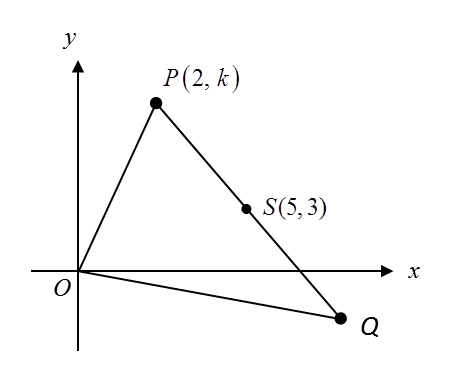\

# 7.8.4 Coordinate Geometry Long Question (Question 7 & 8)

Question 7:
Solutions by scale drawing will not be accepted.
Diagram below shows a triangle OPQ. Point S lies on the line PQ.(a) A point Y moves such that its distance from point S is always 5 uints.
Find the equation of the locus of Y.

(b) It is given that point and point Q lie on the locus of Y    .
Calculate
(i) the value of k,
(ii) the coordinates of Q.

(c) Hence, find the area, in uint2, of triangle OPQ.

Solution:
(a)

(b)(i)
Given P (2, k) lies on the locus of Y.
(2)2 + (k)2– 10(2) – 6(k) + 9 = 0
4 + k2– 20 – 6k + 9 = 0
k2 – 6k – 7 = 0
(k – 7) (k + 1) = 0
k = 7   or   k = – 1
Based on the diagram, k = 7.

(b)(ii)
As P and Q lie on the locus of Y, is the midpoint of PQ. P = (2, 7), S = (5, 3).
Let the coordinates of Q = (x, y),

Coordinates of point Q = (8, –1).

(c)

Question 8:
Diagram below shows a quadrilateral ABCD. Point C lies on the y-axis.The equation of a straight line AD is 2y = 5x – 21
(a) Find
(i) the equation of the straight line AB,
(ii) the coordinates of A,

(b) A point P moves such that its distance from point D is always 5 units.
Find the equation of the locus of P.

Solution:
(a)(i)

(a)(ii)

(b)

### 2 thoughts on “7.8.4 Coordinate Geometry Long Question (Question 7 & 8)”

1.for 7b (ii), how do i know that S is the midpoint of PQ? its not given in the question thanks

•Hi Tye,
It is given in the question that point P and point Q lie on the locus of Y.
PSQ is a straight line and SP = SQ = 5 units, therefore S is the midpoint of PQ.